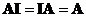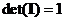## Identity Matrix

Identity matrix is a square matrix whose diagonal elements are equal to one. Non-diagonal elements are zeros.

Identity matrix is fully determined by its order.

Example:The interactive program below will generate identity matrix based on your specified size or order. This program is designed to confirm your basic understanding about identity matrix.

Size =

## Properties

The following are well known properties of identity matrix:

• Identity matrix plays role as multiplicative identity of a square matrix,• The determinant of an identity matrix is one